# BJTs and Logic Gates (basic stuff)

Hello allI am a mechanical engineering student taking an introductory circuits course. I find the things we are doing in lab quite interesting, but I really lack a good understanding of what we are doing. We are currently building logic gates using BJTs. I have some basic questions regarding these. I get the basic idea (I think): We set up a circuit that has some constant source voltage connected to it. Then, if we give it an input voltage of proper magnitude, a *switch* is thrown allowing current to flow from our source voltage to ground. The output is a measured voltage that is either high or low (1 or 0).

I know it really isn't a switch in the mechanical sense of the word, but I don't really want to know about all of the intricacies of doping, so I will just think of it as a switch. Here is the schematic I am looking at of a BJT in such a circuit: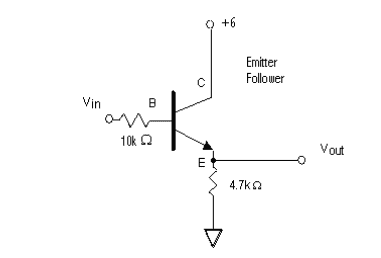Figure 1

Now I really need to clear up what I think is going on here in this circuit (from my reading). The +6V is my constant input voltage connected to the collector C of the transistor. Since the circuit is "open" from C to E, no current can flow and hence the voltage drop from E to ground is zero. Now if we apply the appropriate voltage at B, that *switch* I spoke of is thrown and shorts C to E so that current can now flow. The output is what is labeled here in Figure 1 as Vout, which is measured with respect to ground and should be 6V if the BJT offers no resistance.

Now here is one of my questions that I need to have answered before I continue on to logic gates. The 4.7 kΩ resistor is referred to in my handout as the pull-down resistor.

1) Why is called the "pull-down" resistor?

2) What function(s) does it serve? Why can't we just have a wire from E to ground instead? Is it so the current doesn't go to "infinite"? I am picturing the circuit without that 4.7kΩ resistor as just a voltage source with a wire connecting (+) directly to (-).

I will post logic gate questions once I feel comfortable with this stuff here. Any thoughts are appreciated.

Gold Member
2021 Award
Now here is one of my questions that I need to have answered before I continue on to logic gates. The 4.7 kΩ resistor is referred to in my handout as the pull-down resistor.

1) Why is called the "pull-down" resistor?

Because the Emitter of the transistor is being "Pulled Down" (taken to low) by the resistor

in logic ccts you can also have "pull up" resistors where a particular input or output is pulled hi to the +5V rail via a resistor

2) What function(s) does it serve? Why can't we just have a wire from E to ground instead? Is it so the current doesn't go to "infinite"? I am picturing the circuit without that 4.7kΩ resistor as just a voltage source with a wire connecting (+) directly to (-).

you cant have a short cct from emitter to gnd (0V rail) else the Vout point at the resistor / emitter junction could never be taken hi when then transistor is turned on.
There would basically be a short cct between the + and - rails via the transistors' Collector and Emitter junction

you could do the opposite arrangement, see attached pic....
Now the Vout is being pulled Hi till the transistor is turned on when that point goes low

cheers
Dave

#### Attachments

Last edited:
Because the Emitter of the transistor is being "Pulled Down" (taken to low) by the resistor

in logic ccts you can also have "pull up" resistors where a particular input or output is pulled hi to the +5V rail via a resistor

you cant have a short cct from emitter to gnd (0V rail) else the Vout point at the resistor / emitter junction could never be taken hi when then transistor is turned on.
There would basically be a short cct between the + and - rails via the transistors' Collector and Emitter junction

you could do the opposite arrangement, see attached pic....
Now the Vout is being pulled Hi till the transistor is turned on when that point goes low

cheers
Dave

Hey there DaveThanks for replying. Sorry, but I am a little slow with this stuff.

So "Pull Down" just means the BJT is connected to ground via the resistor and "Pull Up" means it's connected to (+) via the resistor?

And I don't quite understand this

you cant have a short cct from emitter to gnd (0V rail) else the Vout point at the resistor / emitter junction could never be taken hi when then transistor is turned on.

I don't know what you mean by "the Vout point could never be taken hi when the transistor is turned on."

When the transistor is turned on, current flows from source to ground via C-E. Vout is just the point where I put the positive lead of my Volt-meter. So is what you are saying is that I could not measure Vout with respect to ground if there was not a resistor there?

Gold Member
2021 Award
Hey there DaveThanks for replying. Sorry, but I am a little slow with this stuff.
So "Pull Down" just means the BJT is connected to ground via the resistor and "Pull Up" means it's connected to (+) via the resistor?
And I don't quite understand this

yes ... for the sake of arguement swap the "down" and "Up" with 0V and +V
or if you like logic 0 and logic 1
so pulled down to 0V = logic 0

I don't know what you mean by "the Vout point could never be taken hi when the transistor is turned on."

well if the emitter was connected directly to 0V via a wire rather than the resistor
the emitter is at 0V and can only ever be at 0V. you cant develop a voltage potential across a short cct :)

When the transistor is turned on, current flows from source to ground via C-E. Vout is just the point where I put the positive lead of my Volt-meter.

no its not just a measuring point, its the output point of your logic gate, that because of the resistor, is able to be at logic 1 (+6V) or at logic 0 (0V) depending on if the transistor is turned on or not
transistor turned on, its at logic 1 (+6V)
transistor turned off, its at logic 0 (0V)

So is what you are saying is that I could not measure Vout with respect to ground if there was not a resistor there?
correct see earlier comment ... its at 0V potential (short cct'ed to gnd)

Dave

Last edited:
Hi again DaveOk. I will have to chew on this a little more, but i think it is starting to sink in (a little). I think by moving forward with the first of my other questions, it will really click. I am looking at your attached image in post #2. It is a NOT gate if I am not mistaken correct? It looks identical to the image of a NOT gate I have in my lab paper. I don't think I am seeing exactly how this functions.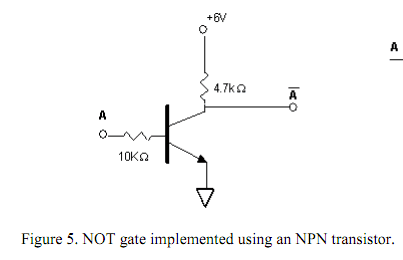We should have a reading of 6V at A_bar when the transistor is off (i.e. when there is no voltage applied at A). I guess I am not see how this happens? Doesn't the 6V drop completely across the 4.7Ω resistor? So wouldn't the voltage at Abar with respect to ground be zero?

Gold Member
davenn is doing a good job explaining, so I don't want to hijack his work here. But I did want to interject that these circuits use http://en.wikipedia.org/wiki/Resistor%E2%80%93transistor_logic" [Broken] (RTL). When you have a logical low, that doesn't mean that you necessarily have 0 volts and when you have a high, that doesn't mean you necessarily have 6 volts (as your source here). In the old days, RTL was used extensively. There is range for a logical low and a range for a logical highs. When the transistor in saturation, the collector to emitter voltage is not 0 volts but low. The same is true for a logical high. A logical high might be lower than your supply voltage.

Last edited by a moderator:
gnurf
Doesn't the 6V drop completely across the 4.7Ω resistor?
With Ohm's Law in mind, what is the voltage drop across the (or any) resistor?

With Ohm's Law in mind, what is the voltage drop across the (or any) resistor?

Meh ... maybe I just won't get this. You guys understand this stuff so well, that when you try to explain it to me, it just isn't dumbed down enough.

I still don't understand what is going on in the very first circuit from post #1. I still don't under stand what the 4.7 kΩ resistor is doing. I said that I did, but I guess I must have been faking itI am going to list all of the assumptions that I have been making about this circuit and hopefully someone will have the patience to correct my bad logic.

1) The +6V is the (+) terminal of our power supply.

2) When the transistor is OFF, nothing is happening. The (-) terminal is not connected to the (+) terminal and so there is no incentive for current to flow. Like a closed dam, it's all just potential.

3) The point labeled Vout is the output and its voltage is the same as that at node E.

4) If we stick the red lead of a voltmeter to node E when the transistor is OFF, the voltmeter reads 0V (ideally) since, since CE is an open circuit the black lead of the voltmeter is always connected to ground where v = 0.

5) When the transistor is ON, we short CE so that current can flow from the +6V terminal to ground.

Is this all correct so far?Gold Member
1) yes.

2) Yes. Note that a real transistor, as opposed to an ideal one, might have a little leakage current but the voltage drop across the 47kω resistor will be low (still a low state)

3) yes.

4) yes.
since CE is an open circuit
See note on 2)

5) No. The transistor will conduct (there will be a collector current), and there is a voltage drop between C and E. So the output won't be 6 volts but (6 - VCE) voltage.

Edit: If you have not done so, now would be a good time to check out http://hyperphysics.phy-astr.gsu.edu/hbase/electric/ohmlaw.html" [Broken].

Last edited by a moderator:
1) yes.

2) Yes. Note that a real transistor, as opposed to an ideal one, might have a little leakage current but the voltage drop across the 47kω resistor will be low (still a low state)

3) yes.

4) yes. See note on 2)

5) No. The transistor will conduct (there will be a collector current), and there is a voltage drop between C and E. So the output won't be 6 volts but (6 - VCE) voltage.

Edit: If you have not done so, now would be a good time to check out http://hyperphysics.phy-astr.gsu.edu/hbase/electric/ohmlaw.html" [Broken].

Hi digoffYes, I have studied Ohm's Law (though it had always pertaied to resistors, but now I see that it applies to many conductors, it just resistors.

Now, just to reiterate what I said in (5) above:

5) When the transistor is ON, we short CE so that current can flow from the +6V terminal to ground.

As you can see, I did not say that the output would be 6V; I was just stating that when the transistor is ON, current flows from the 6V+ terminal to ground. That is correct, is it not?

Last edited by a moderator:
Gold Member
Correct. That would give a voltage ~5.5V (depending how hard you're driving the base since Ic~βIb where β is the transistors gain.

Correct. That would give a voltage ~5.5V (depending how hard you're driving the base since Ic~βIb where β is the transistors gain.

Ok great. I don't know what driving the base means or what gain is, but hopefully it does not matter.

So if the transistor is ON, I have the black lead of my meter at ground and i stick the red lead at E, I should get about ~5.5 V. Correct?

So why do I need the 4.7 kΩ resistor? When CE is closed and current can flow, the voltage measured across the 6V source to ground is the same as the voltage from E to ground (minus the insignificant drop across the transistor).

I don't see the point, let alone the necessity.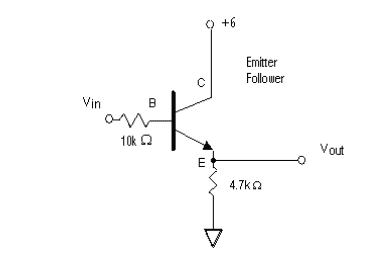Gold Member
So why do I need the 4.7 kΩ resistor? When CE is closed and current can flow, the voltage measured across the 6V source to ground is the same as the voltage from E to ground (minus the insignificant drop across the transistor).

I don't see the point, let alone the necessity.Transistors can only take so much current before you get junction failure (they burn up) withing the transistor. So it does protect the transistor, but really you need it to preform the logic function. If there there weren't a resistor there, there wouldn't be any significant resistance (as opposed to a wire will little resistance) to provide a voltage drop (ohms law) to give a voltage high enough to represent a logical high.

... If there there weren't a resistor there, there wouldn't be any significant resistance (as opposed to a wire will little resistance) to provide a voltage drop (ohms law) to give a voltage high enough to represent a logical high.

Sorry, you guys are going to lose me every time right here. The output Vout occurs before the resistor. How can the resistor possibly effect what voltage is being seen at E?Gold Member
Ground is ground. Without a resistor, you would not be able to use the circuit when Vin high. All the collector current will go to ground providing a Vout that is low; not high as you would want; not to speak of the transistor getting hot and eventually dying for excessive collector current.

When you get into stringing several logic circuit together to preform some desired function, you would want the second stage to see a high or low (depending on the input) from the first gate. If for a 0 or a 1 state you only get a 0 output, then what good is it?

I think it might help to look at what is going on beyond Vout. There is actually stuff connected at Vout. Like in the following image. Now, if we don't use a resistor between E and ground, and instead have a short circuit, there is no incentive for current to flow out into those "other components" which do offer resistance. So in a sense, the resistance is forcing the current to take the Vout path to those "other components."

Am I getting any closer? If not ... I'm going to bed.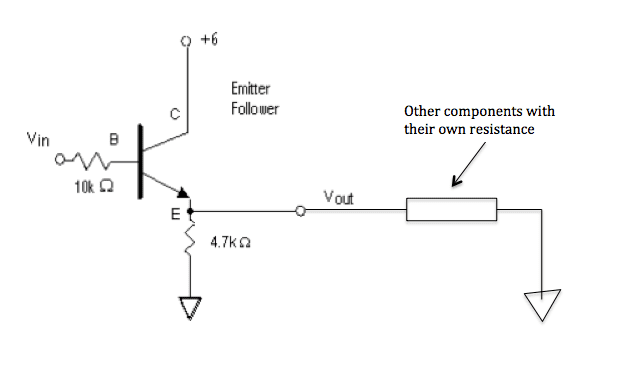You can oversimplify this. The transistor is a BIT like a switch, but it isn't a switch.

In this diagram,
[PLAIN]http://dl.dropbox.com/u/4222062/base%20current.PNG [Broken]

You can see the small red current flowing in from the left. This comes through the 10 K resistor, through the B_E junction of the transistor and down to ground through the 4.7 K resistor.

The flow of this current through the B-E leads of the transistor is what turns the transistor ON.
When it flows, it causes a much larger current (shown as a large red arrow ) to flow.
(incidentally the ratio of these two currents is current gain, so you now know what that is.)

The point is, that if you remove the 4.7K resistor but don't ground the emitter "E", the small current (called base current) cannot flow, so the big current (called the collector current) can't flow either.

If you do ground the emitter,then you ground the output as well, so it can't have any voltage on it.

Last edited by a moderator:
Gold Member
Well the impedance/resistance of the following stage can have a value to put the logical signal in the proper voltage range that the next stage will recognize it in the proper range of a logical O or logical 1. But generally I believe the 4.7kω would be there as a protection element in the event the second stage somehow become unavailable.

You can oversimplify this. The transistor is a BIT like a switch, but it isn't a switch.

If you do ground the emitter,then you ground the output as well, so it can't have any voltage on it.

This is about to reveal how little I understand about electricity: Why? Why does grounding the emitter ground the output as well? And why does that mean that the output cannot have any voltage on it?

This is about to reveal how little I understand about electricity: Why? Why does grounding the emitter ground the output as well? And why does that mean that the output cannot have any voltage on it?

We measure voltages RELATIVE to ground, so if something is joined to ground, it has no voltage relative to ground.

Real wires do have a very small resistance, but for this discussion, it is assumed this resistance is negligible.

Also, when we talk about "ground", we don't mean the soil in your yard. Usually, it just means the negative end of the battery or power supply. This could be connected to real ground or to the mains ground, without affecting the operation of the circuit.

Let's have a little bit of Ohm's Law. This is a deceptively simple law which governs a lot of electrical activities.
The voltage across a resistor depends on the current flowing in it. This may seem obvious, but it is important.
VOLTAGE (in volts) = CURRENT (in Amps) times resistance (in Ohms)

Suppose you have a resistor with 5 amps flowing in it.
If the resistor is 200 ohms, the voltage across the resistor is 1000 volts. (5 times 200 is 1000)
If the resistor is 20 ohms, the voltage across the resistor is 100 volts.
If the resistor is 2 ohms, the voltage across the resistor is 10 volts.

Can you see why a resistor which is just a piece of wire will have almost no voltage across it?
It might have a resistance of only 0.01 ohms, so even a current of 5 amps would produce only 0.05 volts across the piece of wire. This would hardly be visible on your voltmeter.

We measure voltages RELATIVE to ground, so if something is joined to ground, it has no voltage relative to ground.

Real wires do have a very small resistance, but for this discussion, it is assumed this resistance is negligible.

Also, when we talk about "ground", we don't mean the soil in your yard. Usually, it just means the negative end of the battery or power supply. This could be connected to real ground or to the mains ground, without affecting the operation of the circuit.

Let's have a little bit of Ohm's Law. This is a deceptively simple law which governs a lot of electrical activities.
The voltage across a resistor depends on the current flowing in it. This may seem obvious, but it is important.
VOLTAGE (in volts) = CURRENT (in Amps) times resistance (in Ohms)

Suppose you have a resistor with 5 amps flowing in it.
If the resistor is 200 ohms, the voltage across the resistor is 1000 volts. (5 times 200 is 1000)
If the resistor is 20 ohms, the voltage across the resistor is 100 volts.
If the resistor is 2 ohms, the voltage across the resistor is 10 volts.

Can you see why a resistor which is just a piece of wire will have almost no voltage across it?
It might have a resistance of only 0.01 ohms, so even a current of 5 amps would produce only 0.05 volts across the piece of wire. This would hardly be visible on your voltmeter.

I don't see why nobody else can see what it is that I don't understand here. I know Ohm's law. My point is, when the transistor is on, current flows from the +6V terminal from way up top right? It flows through C-E, right? Dropping just a little across C-E. So if it started at +6V relative to ground and then it loses just a little bit across C-E, then that means at E, the voltage is still close to 6V relative to ground. That means that the voltage is still HIGH at point E relative to ground. So I am thinking that if we stick our voltmeter at E and ground we see HIGH voltage.

Or is it that the "ground" is the ground only by virtue of the fact that the resistor IS there to drop the voltage to something sufficiently low enough that we can call it the ground?

Gold Member
2021 Award
This is about to reveal how little I understand about electricity: Why? Why does grounding the emitter ground the output as well? And why does that mean that the output cannot have any voltage on it?

When the emitter is connected directly to 0V it is at that potential... 0V ... it cant be at anything else cuz of the direct connection to the 0V line.
Now when you turn the transistor on you are now directly connecting the +6V line directly to 0V via the collector - emitter junction now you have a short circuit actoss the 6V power supply and as others said earlier the transistor is going to get very hot and probably die quickly.
The 4k7 resistor in the emitter line (leg) to 0V "lifts" the emitter / Vout point above the 0V potential.

Na... no hijack guys :) ya all chimed in whilst I was sleeping through sunday nite and working through monday morn :) thanks for the added input.

I'm not sure how many other ways I can explain this so Saladsamurai finally grasps what is happening :)

ok... forget about the transistor being there for a minute, consider these 3 ccts (A, B, and C) below ....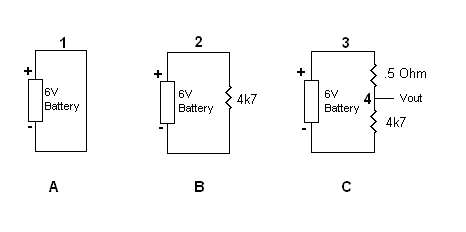what do you think the voltage in cct A will be at point 1
what do you think the voltage in cct B will be at point 2
what do you think the voltage in cct C will be at point 3 and point 4 (Vout)

imagine in cct C the first resistor @ 0.5 Ohms is the transistor junction resistance

explain why you will get the voltage readings you do

Dave

Gold Member
2021 Award

I'm enjoying the session :), as you're not so much testing my knowledge, but my ability, or lack thereof of being able to teach ;) that is to be able to clearly get across an idea to some one. nothing like a good challenge :)

Dave

Gold Member
2021 Award
I don't see why nobody else can see what it is that I don't understand here. I know Ohm's law. My point is, when the transistor is on, current flows from the +6V terminal from way up top right? It flows through C-E, right? Dropping just a little across C-E. So if it started at +6V relative to ground and then it loses just a little bit across C-E, then that means at E, the voltage is still close to 6V relative to ground. That means that the voltage is still HIGH at point E relative to ground. So I am thinking that if we stick our voltmeter at E and ground we see HIGH voltage.

ok another way.....

look at this cct in ref to the above quote from you....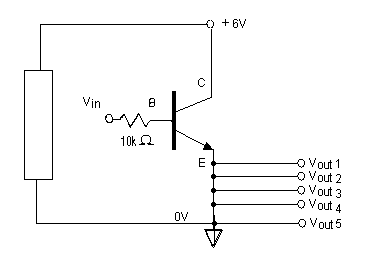WITH transistor turned off.....
negative lead of meter on 0V line from battery... now with positive lead of meter, measure the voltage at Vout 1 through to Vout 5 what are you going to read ?

and why ?

WITH transistor turned on.....
negative lead of meter on 0V line from battery... now with positive lead of meter, measure the voltage at Vout 1 through to Vout 5 what are you going to read ?

and Why ?

Dave

I don't see why nobody else can see what it is that I don't understand here. I know Ohm's law. My point is, when the transistor is on, current flows from the +6V terminal from way up top right? It flows through C-E, right? Dropping just a little across C-E. So if it started at +6V relative to ground and then it loses just a little bit across C-E, then that means at E, the voltage is still close to 6V relative to ground. That means that the voltage is still HIGH at point E relative to ground. So I am thinking that if we stick our voltmeter at E and ground we see HIGH voltage.

Or is it that the "ground" is the ground only by virtue of the fact that the resistor IS there to drop the voltage to something sufficiently low enough that we can call it the ground?

You have one lead of your multimeter on the ground and one lead on E. In between these, you propose placing a piece of wire with a very low resistance.
Now, the only way this can have a voltage across it is to send a very large current through it.
This is Ohms Law.

And a very large current is not available. The current from the emitter will be at most about 50 mA if the transistor has a gain of 100. This is another reason the transistor is not like a mechanical switch. The current is controlled.

When the emitter is connected directly to 0V it is at that potential... 0V ... it cant be at anything else cuz of the direct connection to the 0V line.
Now when you turn the transistor on you are now directly connecting the +6V line directly to 0V via the collector - emitter junction now you have a short circuit actoss the 6V power supply and as others said earlier the transistor is going to get very hot and probably die quickly.
The 4k7 resistor in the emitter line (leg) to 0V "lifts" the emitter / Vout point above the 0V potential.

Na... no hijack guys :) ya all chimed in whilst I was sleeping through sunday nite and working through monday morn :) thanks for the added input.

I'm not sure how many other ways I can explain this so Saladsamurai finally grasps what is happening :)

Dave

I know, I know guys ... I suck. Haha! And FYI, as infuriating as this is (my lack of understanding), I really am enjoying this too! This is how I learn something new. I struggle through it. I am not gifted by any means (hence the high post count consisting of all questions like this). But in the course of the struggle, I pick up so much more information through these interactions than I ever could through a text. I could just as easily accept all of what you are saying at face value and go ahead and build some circuits in the lab and be done with it. But I think there is something more to be had from the learning process.

Ok ... back on topic. Let's discuss this part, since this exact image has crossed my mind during our discourse (added some labels to the image):

ok... forget about the transistor being there for a minute, consider these 3 ccts (A, B, and C) below ....

what do you think the voltage in cct A will be at point 1
what do you think the voltage in cct B will be at point 2
what do you think the voltage in cct C will be at point 3 and point 4 (Vout)

imagine in cct C the first resistor @ 0.5 Ohms is the transistor junction resistance

explain why you will get the voltage readings you do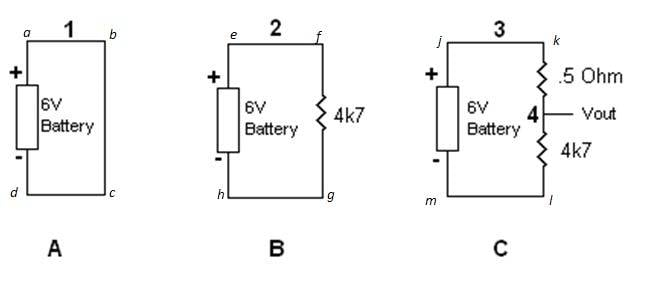In Image A:
At point 1 I would have to say that the voltage is 6V relative to the negative terminal. If we start at point d and travel upward through the loop, there is a voltage rise of 6V and then no sink. So at every point in the wire, the voltage is 6V.

In Image B:
At point 2, again the voltage is 6V. If we start at point h and travel upward through the loop, there is a voltage rise of 6V and then no sink before point 2. So any point in the wire from the + terminal to the resistor has a voltage of 6V.

In Image C:
At point 3, same deal of 6V for the same reason, start at point m and travel upward through the loop, there is a voltage rise of 6V and then no sink before point 3. At point 4 (Vout) the voltage would be 6V - I1R1 or from Ohm's Law Vout = 6V - 6V*R1/(R1+R2).

Gold Member
2021 Award
Ok ... back on topic. Let's discuss this part, since this exact image has crossed my mind during our discourse (added some labels to the image):

In Image A:
At point 1 I would have to say that the voltage is 6V relative to the negative terminal. If we start at point d and travel upward through the loop, there is a voltage rise of 6V and then no sink. So at every point in the wire, the voltage is 6V.

Ahhh No :) --- its a wire straight from the + to the - terminal of the battery, a short circuit, Voltage will be 0V, current will be maximum.
try it with a really small battery say a 1.5V AA battery or a 6V lantern battery
put your multimeter across the terminals read the voltage 1.5V or 6V whatever the battery
now VERY briefly short out the battery terminals and watch the voltage drop to 0V.
Please dont do it on a car battery, even briefly it can supply massive short cct current

This is the bit you need to understand to see the picture in what is happening in the transistor cct if the 4k7 resistor isnt in the circuit between the Emitter and the 0V line
The transistor junction, with only a VERY tiny C-E resistance is basically a short cct across the +6V power supply.

In Image B:
At point 2, again the voltage is 6V. If we start at point h and travel upward through the loop, there is a voltage rise of 6V and then no sink before point 2. So any point in the wire from the + terminal to the resistor has a voltage of 6V.

correct

In Image C:
At point 3, same deal of 6V for the same reason, start at point m and travel upward through the loop, there is a voltage rise of 6V and then no sink before point 3. At point 4 (Vout) the voltage would be 6V - I1R1 or from Ohm's Law Vout = 6V - 6V*R1/(R1+R2).

Correct
Total current flow in cct It = V / R1 + R2 = 6V / 4700 Ohms + 0.5 Ohms =
6V / 4700.5 = 1mA
So voltage drop across 0.5 Ohm resistor is V = I x R = 0.001A x 0.5 Ohms = 0.0005 V

But that low V drop is only cuz of the presence of the 4k7 resistor, which is limiting the current flow through the cct to 1mA

Dave